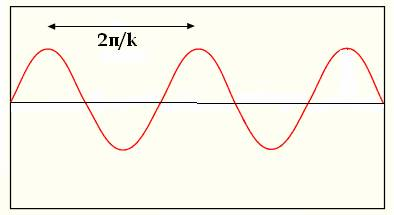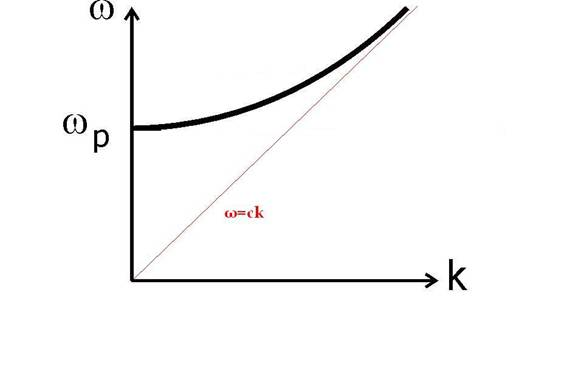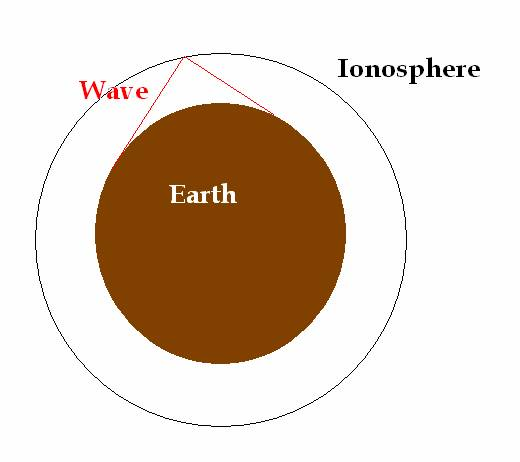TRANSVERSE WAVES IN PLASMAS

Let’s start by remembering what we know about waves in general, such as a wave on a guitar string. We know that the displacement of the string from its equilibrium position is proportional to sin(kx-ωt), as shown belowThe relation between the frequency ω and the wavenumber k is called “dispersion relation” and is characteristic of the medium, where the wave propagates. For E/M waves in vacuum we know that ω=ck.

So, what happens if we try to send a transverse electromagnetic wave through a plasma?

First we have to define the properties of the plasma!

First of all, it is an electron-ion plasma with equal density (so it is overall neutral)

Because of their high mass, we consider the ions as static, so everything is determined by the motion of the electrons.

There is no constant magnetic field present (unmagnetized plasma) and we neglect the thermal motion of the electrons (cold plasma).

Under the above assumptions, the dispersion relation that can be calculated is presented in the figure belowLet’s see what this means!

First of all, we see that as the frequency gets higher, the graph tends to become the vacuum relation ω=ck. Is this understandable?

Of course, this should be anticipated. Due to their inertia, as the changes in the field happen faster and faster, the electron become unable to follow them, so the wave goes through plasma as if it weren’t there!

On the other end, we see that there is nothing below, a certain value, which is our old friend the Plasma frequency!

What happens there? Any wave that is below that frequency cannot propagate in the plasma, and is therefore REFLECTED.

Sounds familiar? Well, it should. Remember the old days, when people used to bounce radiowaves off the ionosphere? That’s how they did it! If you use a frequency that is lower than the plasma frequency for the ionospheric plasma, the radiowaves will be reflected. That way, one can beat the curvature of the earth and transmit signals in large distances.However nowadays we have satellites, so we do not want the ionosphere to reflect our signals!

Easy again! We use a high enough frequency, so that the wave practically ignores the existence of the ionospheric plasma!

We see that with a simplified model, we can understand a lot about modern telecommunications!